# Read, Analyze and Process SOFA Files

SOFA (Spatially Oriented Format for Acoustics)  is a file format for storing spatially oriented acoustic data like head-related transfer functions (HRTF) and binaural or spatial room impulse responses. SOFA has been standardized by the Audio Engineering Society (AES) as AES69-2015.

In this example, you load a SOFA file containing HRTF measurements for a single subject in MATLAB. You then analyze the HRTF measurements in the time domain and the frequency domain. Finally, you use the HRTF impulse responses to spatialize an audio signal in real time by modeling a moving source based on desired azimuth and elevation values.

### Explore a SOFA File in MATLAB

You use a SOFA file from the SADIE II database . The file corresponds to spatially discrete free-field in-the-ear HRTF measurements for a single subject. The measurements characterize how each ear receives a sound from a point in space.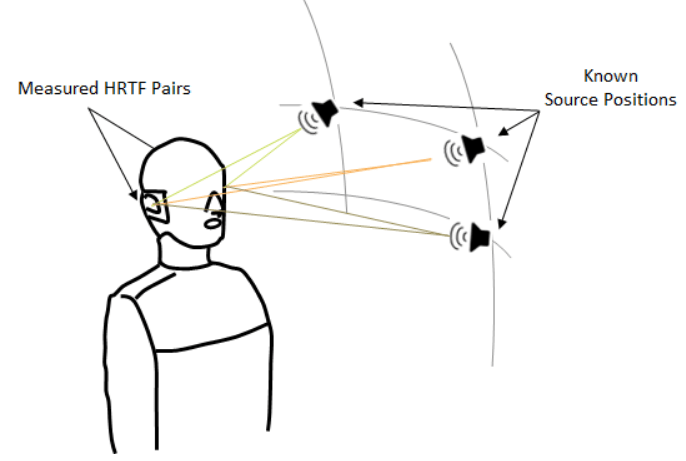```downloadFolder = matlab.internal.examples.downloadSupportFile("audio","SOFA/SOFA.zip"); dataFolder = tempdir; unzip(downloadFolder,dataFolder) netFolder = fullfile(dataFolder,"SOFA"); addpath(netFolder) filename = "H10_48K_24bit_256tap_FIR_SOFA.sofa";```

#### Display SOFA File Contents

SOFA files consist of binary data stored in the netCDF-4 format. You can use MATLAB to read and write netCDF files.

Display the contents of the SOFA file using `ncdisp` (execute `ncdisp(filename)`).

The file contents consist of multiple fields corresponding to different aspects of the measurements, such as the (fixed) listener position, the varying source position, the coordinate system used to capture the data, general metadata related to the measurement, as well as the measured impulse responses.

NetCDF is a "self-describing" file format, where data is stored along with attributes that can be used to assist in its interpretation. Consider the display snippet corresponding to the source position for example: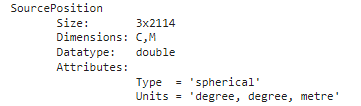`SourcePosition` contains the coordinates for the varying source position used in the measurements (here, there are 2114 separate positions). The file also contains attributes (Type, Units) describing the coordinate system used to store the positions (here, spherical), as well as information about the dimensions of the data (C,M). The dimensions are defined in the AES69 standard :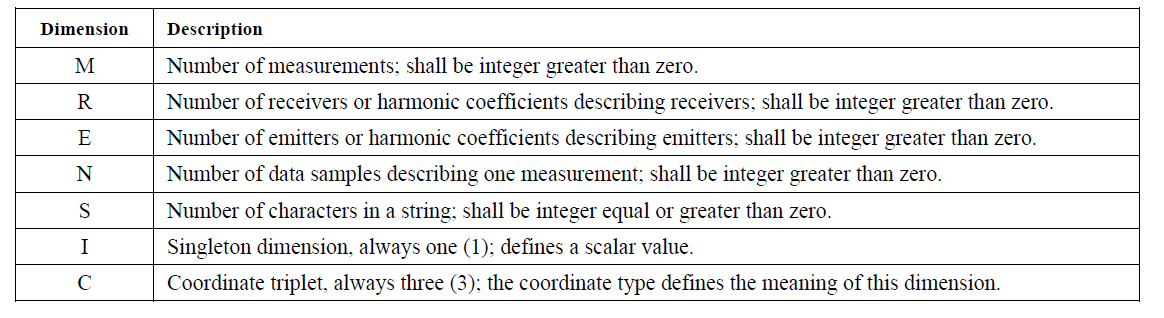For the file in this example:

• M = 2114 (the total number of measurements, each corresponding to a unique source position).

• R = 2 (corresponding to the two ears).

• E = 1 (one emitter or sound source per measurement).

• N = 256 (the length of each recorded impulse response).

Use `ncinfo` to get information about the SOFA file.

`SOFAInfo = ncinfo(filename);`

The fields of the structure `SOFAInfo` hold information related to the file's dimensions, variables and attributes.

#### Read a Variable from the SOFA File

Use `ncread` to read the value of a variable in the SOFA file.

Read the variable corresponding to the measured impulse responses.

```ir = ncread(filename,"Data.IR"); size(ir)```
```ans = 1×3 256 2 2114 ```

This variable holds impulse responses for the left and right ear for 2114 independent measurements. Each impulse response is of length 256.

Read the sampling rate of the measurements.

`fs = ncread(filename,"Data.SamplingRate")`
```fs = 48000 ```

Plot the first measured impulse response.

```figure; t = (0:size(ir,1)-1)/fs; plot(t,ir(:,1,1)) grid on xlabel("Time (s)") ylabel("Impulse response")```It is possible to read and analyze the contents of the SOFA file using a combination of `ncinfo` and `ncread`. However, the process can be cumbersome and time consuming.

The function `sofaread` allows you to read the entire contents of a SOFA file in one line of code.

Use `sofaread` to get the contents of the SOFA file.

`s = sofaread(filename)`
```s = audio.sofa.SimpleFreeFieldHRIR with properties: Numerator: [2114×2×256 double] Delay: [0 0] SamplingRate: 48000 SamplingRateUnits: "hertz" Show all properties ```

Click "Show all properties" in the display above to see the rest of the properties from the SOFA file.

You can easily access the measured impulses responses.

```ir = s.Numerator; size(ir)```
```ans = 1×3 2114 2 256 ```

You access other variables in a similar fashion. For example, read the source positions along with the coordinate system used to express them:

```srcPositions = s.SourcePosition; size(srcPositions)```
```ans = 1×2 2114 3 ```
`srcPositions(1,:)`
```ans = 1×3 0 -90.0000 1.2000 ```
`s.SourcePositionType`
```ans = 'spherical' ```
`s.SourcePositionUnits`
```ans = "degree, degree, meter" ```

### View the Measurement Geometry

Call the helper function `plotGeometry` to view the general geometric setup of the measurements.

```figure; plotGeometry(s) a = gca; a.CameraPosition = [-3 -16 12];```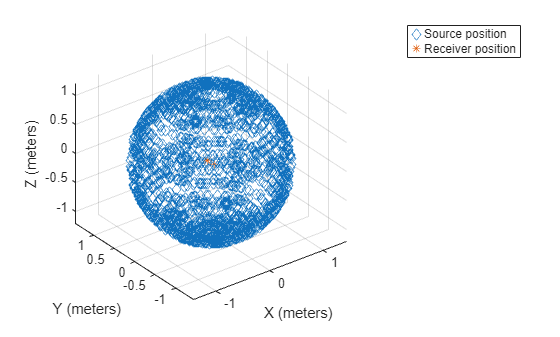Alternatively, specify input indices to restrict the plot to desired source locations.

```figure; plotGeometry(s,1:100) a = gca; a.CameraPosition = [-3 -16 12];```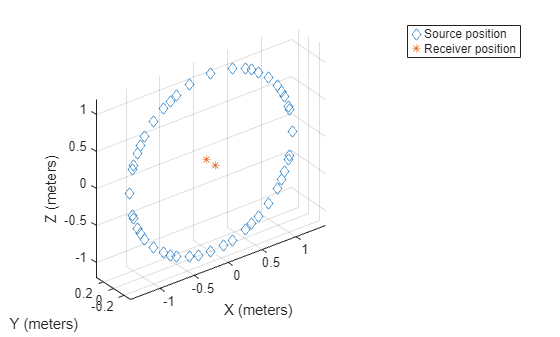### Compute HRTF Frequency Responses

The file in this example uses the `SimpleFreeFieldHRIR` convention, which stores impulse response measurements in the time domain as FIR filters.

`s.SOFAConventions`
```ans = "SimpleFreeFieldHRIR" ```
`s.DataType`
```ans = "FIR" ```

It is straightforward to compute and plot the frequency response of the impulse responses using `freqz`.

As an illustration, assume you want to plot the frequency response of measurements with an azimuth value in the range of 30 degrees to 32 degrees.

First, inspect the type of the source position data.

`s.SourcePositionType`
```ans = 'spherical' ```
`s.SourcePositionUnits`
```ans = "degree, degree, meter" ```

The coordinates are spherical, which is convenient for your purpose. Also, note that the units are in degrees, so conversion from radians per second to degrees in not necessary.

Per the SOFA standard , the first angle value corresponds to the azimuth.

`az = s.SourcePosition(:,1);`

Find the values corresponding to the desired azimuth range.

`indices = find(az>30 & az<32);`

You are interested in the impulse responses corresponding to the first receiver (ear) only. Read the corresponding impulse responses.

```receiver = 1; IR = s.Numerator(indices,receiver,:); IR = permute(IR,[3 1 2]); IR = squeeze(IR); size(IR)```
```ans = 1×2 256 22 ```

Use `freqz` to compute the frequency response of each FIR filter. Specify a 4096-point frequency response.

```N = 4096; H = zeros(N,length(indices)); for index = 1:length(indices) [H(:,index),F] = freqz(IR(:,index),1, N ,s.SamplingRate); end```

Plot the magnitude responses.

```figure for index=1:length(indices) plot(F,20*log10(abs(H(:,index)))); hold on end grid on ylabel("Magnitude (dB)"); xlabel("Frequency (Hz)")```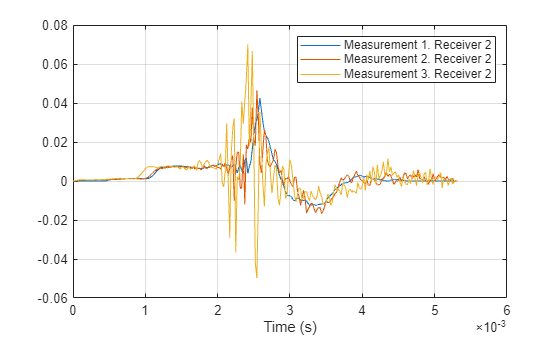### Compute HRTF Spectra

It is often useful to compute and visualize the magnitude spectra of HRTF data in a specific plane in space.

For example, compute the spectrum in the horizontal plane (corresponding to an elevation angle equal to zero) for the first receiver.

Find measurements with an elevation angle within 2 degrees of zero.

```threshold = 2; ele = s.SourcePosition(:,2); indices = find(abs(ele)<threshold); ```

Read the corresponding impulse responses for one ear.

```receiver = 1; IR = s.Numerator(indices,receiver,:); IR = permute(IR,[3 1 2]); IR = squeeze(IR); size(IR)```
```ans = 1×2 256 96 ```

Use `freqz` to compute the frequency response of each FIR filter. Specify a 4096-point frequency response.

```N = 4096; H = zeros(N,length(indices)); for index = 1:length(indices) [H(:,index),F] = freqz(IR(:,index),1, N ,s.SamplingRate); end```

Convert to log scale.

`H = 20*log10(abs(H)).';`

Eliminate small values by using a noise floor.

```noiseFloor = -50; H(H<noiseFloor) = noiseFloor;```

Sort data by azimuth values. Note that the SOFA standard uses an azimuth range of [0, 360] degrees. Convert it to [-180,180] degrees.

```azi = s.SourcePosition(indices,1); azi(azi>180)=azi(azi>180)-360; [azi,ind]=sort(azi); H = H(ind,:);```

Plot the horizontal plane spectrum.

```figure surface(F.',azi,H(:,:)); shading flat xlabel("Frequency (Hz)"); ylabel("Azimuth (deg)"); colorbar; axis tight```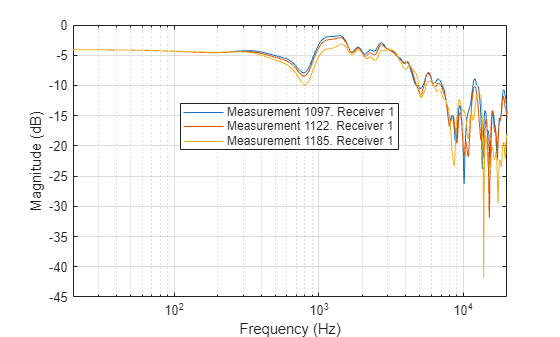### Compute Energy-Time Curve

It is often useful to visualize the decay of the HRTF responses over time using an energy-time curve (ETC).

In this section, you measure and plot the ETC in the horizontal plane. You use the same impulse responses you used to compute the magnitude spectrum in the horizontal plane in the previous section.

Convert the impulse response values to the log domain.

`IRLog = 20*log10(abs(IR));`

Similar to the previous section, sort the data by azimuth values.

`IRLog = IRLog(:,ind);`

Eliminate values smaller than the noise floor.

```noiseFloor=-50; IRLog(IRLog<=noiseFloor)=noiseFloor;```

Plot the ETC.

```fs = s.SamplingRate; t = 0:1/fs*1000:(size(IRLog,1)-1)/fs*1000; figure surface(t,azi,IRLog.'); set(gca,FontName="Arial",FontSize=10); set(gca, TickLength=[0.02 0.05]); set(gca,LineWidth=1); cmap=colormap(hot); cmap=flipud(cmap); shading flat colormap(cmap); box on; colorbar; xlabel("Time (ms)"); ylabel("Azimuth (deg)"); axis tight grid on```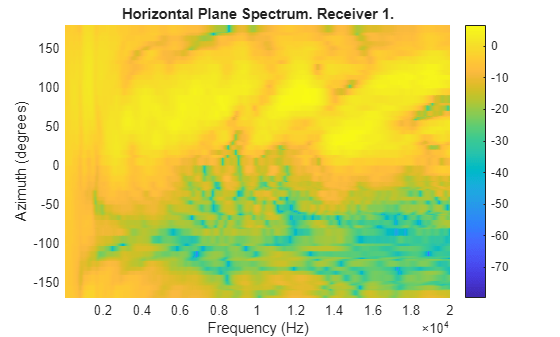### Compute Interaural Time Delay

Interaural time delay (ITD) is the difference in arrival time of a sound between two ears. It is an important binaural cue for sound source localization. There are many ITD estimation methods (see ). Here, you compute the ITD using a simple cross-correlation technique.

First, pass the impulse responses through a lowpass filter.

Design the lowpass filter.

```cutOffFreq = 3000; fs = s.SamplingRate; cutOffFreqNorm = cutOffFreq/(fs/2); [b,a] = butter(5,cutOffFreqNorm);```

Filter the impulse responses.

`ir = filter(b,a,s.Numerator,[],3);`

Estimate the delay between the left and right ear responses using a cross-correlation metric.

```pos = size(ir,1); toa_diff = zeros(1,pos); N = size(ir,3); for ii=1:pos cc = xcorr(squeeze(ir(ii,1,:)),squeeze(ir(ii,2,:))); [~,idx_lag] = max(abs(cc)); toa_diff(ii) = idx_lag - N; end toa_diff = toa_diff/fs;```

Plot ITD versus the azimuth angle in the horizontal plane using the helper function `horizontalITD`. The method leverages `polarplot`.

`horizontalITD(s,toa_diff)`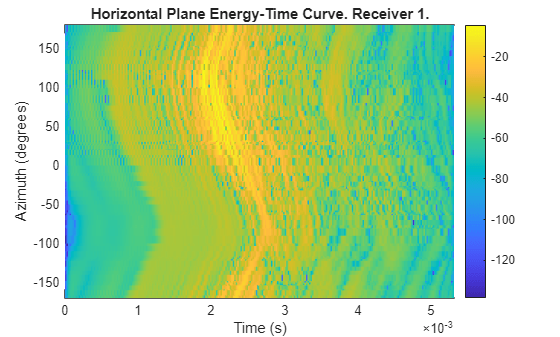### Interpolate HRTF Measurements

The HRTF measurements in the SOFA file correspond to a finite number of azimuth/elevation angle combinations. It is possible to interpolate the data to any desired spatial location using 3-D HRTF interpolation with `interpolateHRTF`.

Specify the desired source position (in degrees).

```desiredAz = [-120;-60;0;60;120;0;-120;120]; desiredEl = [-90;90;45;0;-45;0;45;45]; desiredPosition = [desiredAz, desiredEl];```

Calculate the head-related impulse response (HRIR) using the vector base amplitude panning interpolation (VBAP) algorithm at a desired source position.

```interpolatedIR = interpolateHRTF(s, ... desiredPosition);```

#### Model Moving Source Using HRIR Filtering

Filter a mono input through the interpolated impulse responses to model a moving source.

Create an audio file sampled at 48 kHz for compatibility with the HRTF dataset.

```desiredFs = s.SamplingRate; [x,fs] = audioread("Counting-16-44p1-mono-15secs.wav"); y = audioresample(x,InputRate=fs,OutputRate=desiredFs); y = y./max(abs(y)); audiowrite("Counting-16-48-mono-15secs.wav",y,desiredFs);```

Create a `dsp.AudioFileReader` object to read in a file frame by frame. Create an `audioDeviceWriter` object to play audio to your sound card frame by frame. Create a `dsp.FrequencyDomainFIRFilter` object. Set the `Numerator` property to the combined left-ear and right-ear filter pair. Since you want to keep the received signals at each ear separate, set `SumFilteredOutputs` to false.

```fileReader = dsp.AudioFileReader("Counting-16-48-mono-15secs.wav"); deviceWriter = audioDeviceWriter(SampleRate=fileReader.SampleRate); spatialFilter = dsp.FrequencyDomainFIRFilter(squeeze(interpolatedIR(1,:,:)),SumFilteredOutputs=false);```

In an audio stream loop:

1. Read in a frame of audio data.

2. Feed the audio data through the filter.

3. Write the audio to your output device. If you have a stereo output hardware, such as headphones, you can hear the source shifting position over time.

4. Modify the desired source position in 2-second intervals by updating the filter coefficients.

```durationPerPosition = 2; samplesPerPosition = durationPerPosition*fileReader.SampleRate; samplesPerPosition = samplesPerPosition - rem(samplesPerPosition,fileReader.SamplesPerFrame); sourcePositionIndex = 1; samplesRead = 0; while ~isDone(fileReader) audioIn = fileReader(); samplesRead = samplesRead + fileReader.SamplesPerFrame; audioOut = spatialFilter(audioIn); deviceWriter(audioOut); if mod(samplesRead,samplesPerPosition) == 0 sourcePositionIndex = sourcePositionIndex + 1; spatialFilter.Numerator = squeeze(interpolatedIR(sourcePositionIndex,:,:)); end end```

As a best practice, release your System objects when complete.

```release(deviceWriter) release(fileReader) release(spatialFilter);```

### Spatialize Audio in Real Time

Simulate a sound source moving in the horizontal plane, with an initial azimuth of -90 degrees. Gradually increase the azimuth as the simulation is running.

Compute the starting impulse responses based on the initial source position.

```index = 1; loc = [-90 0]; interpolatedIR = interpolateHRTF(s, ... loc); spatialFilter.Numerator = squeeze(interpolatedIR);```

Execute the simulation loop. Shift the source elevation by 30 degrees every 100 loop iterations. Use `interpolateHRTF` to estimate the new desired impulse responses.

```while ~isDone(fileReader) index=index+1; frame = fileReader(); frame = frame(:,1); audioOut = spatialFilter(frame); deviceWriter(audioOut); if mod(index,100)==0 loc(1)=loc(1)+30; interpolatedIR = interpolateHRTF(s, ... loc); spatialFilter.Numerator = squeeze(interpolatedIR); end end```
```release(deviceWriter) release(fileReader) release(spatialFilter);```

### Helper Functions

```function plotGeometry(s,varargin) % PLOTGEOMETRY Plot measurement geometry of SOFA data % plotGeometry(s) plots the measurement geometry, including the % receiver position, receiver orientation, and source % positions. s is a SOFA object returned by sofaread. % % plotGeometry(s,ind) restricts the plot to the source % positions corresponding to indices in the vector ind. numMeas = size(s.SourcePosition); if nargin ==1 ind = 1:numMeas; else ind = varargin{1}; end figure; hold on; LP = convertCoordinates(s.ListenerPosition,s.ListenerPositionType,"cartesian"); RP = convertCoordinates(s.ReceiverPosition,s.ReceiverPositionType,"cartesian"); SP = convertCoordinates(s.SourcePosition(ind,:),s.SourcePositionType,"cartesian"); LV = convertCoordinates(s.ListenerView,s.ListenerViewType,"cartesian"); if isprop(s,'ListenerUp') try LU = convertCoordinates(s.ListenerUp,s.ListenerUpType,"cartesian"); catch % if ListenerUpType is not defined, try listenerViewType LU = convertCoordinates(s.ListenerUp,s.ListenerViewType,"cartesian"); end end legendEntries = []; legendEntries(1) = plot3(LP(:,1),LP(:,2),LP(:,3),"ro",MarkerFaceColor="r",MarkerSize=5); legendEntries(2) = plot3(LP(1,1)+RP(1,1), LP(1,2)+RP(1,2), LP(1,3)+RP(1,3),"r*",MarkerSize=8); plot3(LP(1,1)+RP(2,1), LP(1,2)+RP(2,2), LP(1,3)+RP(2,3),"r*",MarkerSize=8); legendEntries(3) = plot3(SP(:,1),SP(:,2),SP(:,3),"bd",MarkerSize=7); legendEntries(4) = quiver3(LP(1,1),LP(1,2),LP(1,3),LV(1,1),LV(1,2),LV(1,3),Color=[1 0 0],MarkerFaceColor=[1 0 0]); legendEntries(5) = quiver3(LP(1,1),LP(1,2),LP(1,3),LU(1,1),LU(1,2),LU(1,3),0,AutoScale="off",Color=[0 0 0],MarkerFaceColor=[0 0 0]); legend(legendEntries,["ListenerPosition","ReceiverPosition","SourcePosition","ListenerView","ListenerUp"],Location="NorthEastOutside"); xlabel(["X / " s.ListenerPositionUnits]); ylabel(["Y / " s.ListenerPositionUnits]); zlabel(["Z / " s.ListenerPositionUnits]); axis tight grid on end function output = convertCoordinates(input,input_type,output_type,~,~) % convertCoordinates - Convert between the coordinates systems output=input; % convert to Cartesian if necessary if strcmp(output_type,input_type)==0 temp=input; switch input_type case "cartesian" %do nothing case {"spherical","geodesic"} [temp(:,1),temp(:,2),temp(:,3)]=sph2cart(deg2rad(input(:,1)),deg2rad(input(:,2)),input(:,3)); end output=temp; switch output_type case "cartesian" %do nothing case {"spherical","geodesic"} [output(:,1),output(:,2),output(:,3)]=cart2sph(temp(:,1),temp(:,2),temp(:,3)); output(:,1:2)=rad2deg(output(:,1:2)); end end end function horizontalITD(obj,itd,varargin) % HORIZONTALITD Plot ITD in horizontal plane % horizontalitd(s) plots the inter-aural time delay in the % horizontal plane % % horizontalitd(s, Threshold=TH) specifies the threshold % (in degrees) used to determine measurements belonging to the % horizontal plane. p = inputParser; p.addParameter("Threshold",2); p.parse(varargin{:}); r = p.Results; threshold = r.Threshold; pos = obj.SourcePosition; idx=find(pos(:,2)<threshold & pos(:,2)>-threshold); itd = itd(idx); [pos, idx_sort] = sort(pos(idx,1)); itd = itd(idx_sort); angles = deg2rad(pos); itd = abs(itd); polarplot(angles, itd, linewidth= 1.2,Marker="*"); ax = gca; ax.ThetaDir = "counterclockwise"; ax.ThetaZeroLocation = "top"; rticks([max(itd)*2/3, max(itd)]); rticklabels({[num2str(round(max(itd)*2/3*1e6,1)) ' ' char(181) 's'],... [num2str(round(max(itd)*1e6,1)) ' ' char(181) 's']}); thetaticks(0:30:330) thetaticklabels({'0°', '30°', '60°', '90°', '120°', '150°', '180°', ... '210°', '240°','270°', '300°', '330°'}); grid on; end```

### References

 Majdak, P., Zotter, F., Brinkmann, F., De Muynke, J., Mihocic, M., and Noisternig, M. (2022). “Spatially Oriented Format for Acoustics 21: Introduction and Recent Advances,” J Audio Eng Soc 70, 565–584. DOI: 10.17743/jaes.2022.0026.

 Majdak, P., De Muynke, J., Zotter, F., Brinkmann, F., Mihocic, M., and Ackermann, D. (2022). AES standard for file exchange - Spatial acoustic data file format (AES69-2022), Standard of the Audio Engineering Society. https://www.aes.org/publications/standards/search.cfm?docID=99

 Andreopoulou A, Katz BFG. Identification of perceptually relevant methods of inter-aural time difference estimation. J Acoust Soc Am. 2017 Aug;142(2):588. doi: 10.1121/1.4996457. PMID: 28863557.